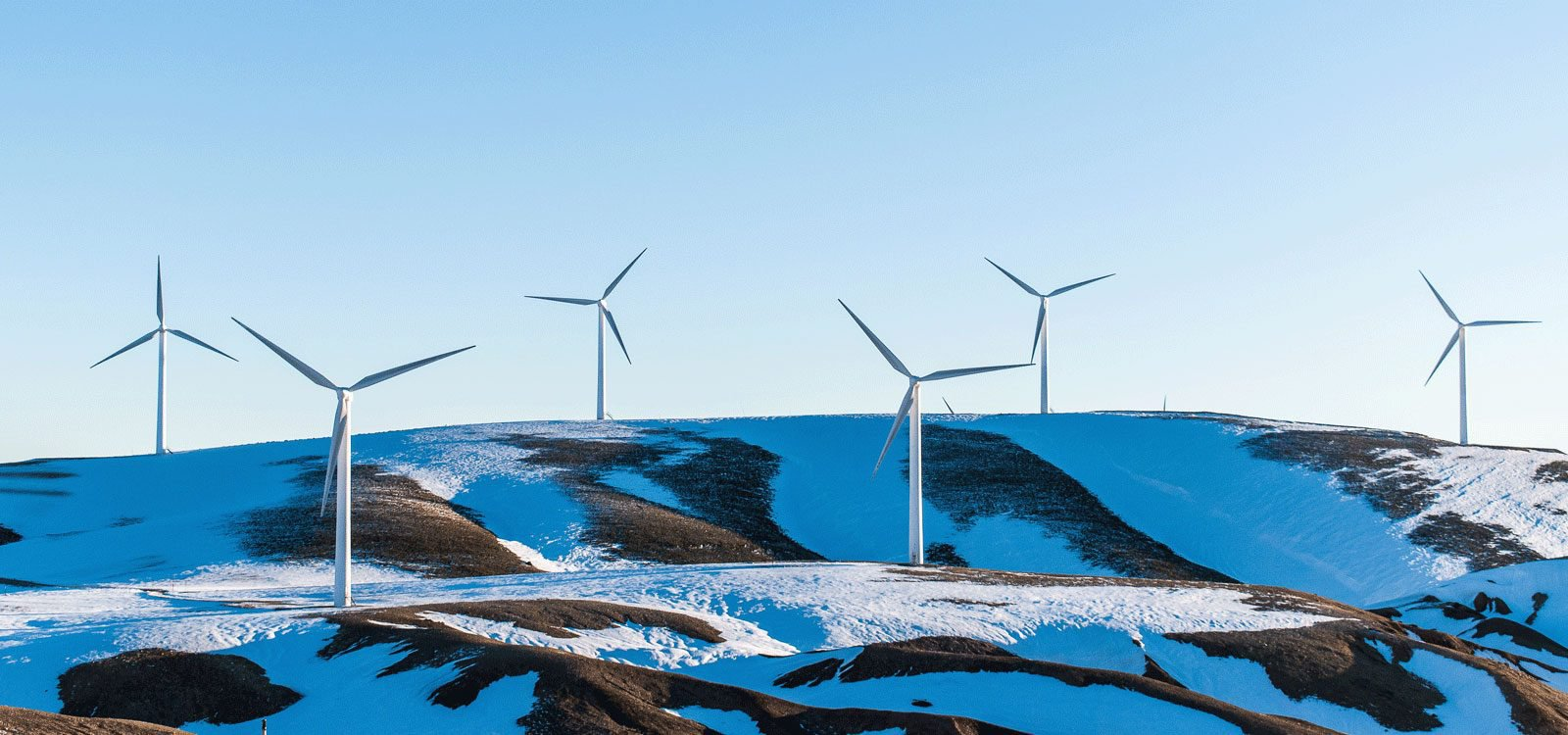Update to Wind Power Normal AssumptionsProduct
Jan. 24, 2019

Advances in wind power technology will give us windmills with increased power efficiency. We are now taking this development into account when computing normal assumptions for wind power.

Update 14.06.19
The update has now been implemented for all other remaining areas as well. (Original post follows)

When we compute the wind production several years ahead (i.e. normal assumptions), it is very clear that the production is heavily affected by the development of wind power capacity (see figure below). There is also another factor that should be taken into account when calculating normal assumptions, and that is the increased efficiency of future wind mills. Windmills in the future will be able to produce more power from the same amount of wind.

This last factor of increased efficiency has traditionally not been a part of our model, but this is about to change. We are now multiplying the production with an efficiency factor. This factor will typically increase the further ahead in time we are predicting and lead to increased normal assumptions.

The result can be seen below. Notice how the normal assumptions have increased after being multiplied with an efficiency factor. The wind power capacity is also plotted, to display its close relation to the normal assumptions.For now, this update has only been implemented for the Nordic areas (NO, SE, DK), but it is going to be implemented for the other areas as well.

Andreas Malmgård